Vectors and conics

This free course is available to start right now. Review the full course description and key learning outcomes and create an account and enrol if you want a free statement of participation.

Free course

1.2 Lines

The equation of any line in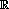2, except a line parallel to the y-axis, can be written in the form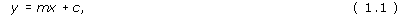where m is the gradient or slope of the line, and c is its y-intercept; that is, (0, c) is the point at which the line crosses the y-axis. (See the first sketch below.)

In the particular case that the line cuts the y-axis at the origin, its equation has the simple form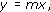as c = 0 in this case. (See the second sketch below.)

Another special case occurs when m = 0. Then the line is parallel to the x-axis, and its equation is of the form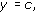where c is the y-intercept. (See the third sketch below.)

Finally, the equation of a line parallel to the y-axis cannot be written in the form y = mx + c, but it can be written as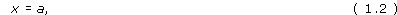where (a, 0) is the point at which the line crosses the x-axis. (See the final sketch below.)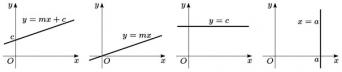In both cases (1.1) and (1.2) above, the equation of a line in the plane can be written in the form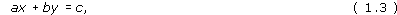for some real numbers a, b and c, where a and b are not both zero.

Thus any line in2 has an equation of the form (1.3); conversely, any equation of the form (1.3) represents a line in2.

Equation of a line

The general equation of a line in2 is

ax + by = c,

where a, b and c are real, and a and b are not both zero.

Exercise 1

Determine the equation of the line with gradient −3 that passes through the point (2, −1).

Using the formula for the equation of a line when given its gradient and one point on it, we find that the equation of this line is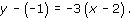We can rearrange this in the form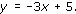Exercise 2

For each of the following pairs of points, determine the equation of the line through them.

• (a) (1, 1) and (3, 5).

• (b) (0, 0) and (0, 8).

• (c) (0, 0) and (4, 2).

• (d) (4, −1) and (2, −1).

(a) Since (1, 1) and (3, 5) lie on the line, its gradient is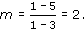Then, since the point (1, 1) lies on the line, its equation must be y − 1 = 2(x − 1), or y = 2x − 1.

(b) Both these points have x-coordinate 0, so they lie on the line with equation x = 0, the y-axis.

(c) Since the origin lies on the line, its equation must be of the form y = mx, where m is its gradient.

Since (4, 2) lies on the line, its coordinates must satisfy the equation of the line. Thus 2 = 4 m, so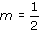Hence the equation of this line is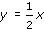(d) Both these points have y-coordinate −1, so they lie on the line with equation y = −1.

M208_1